# 選擇排序法（Selection Sort）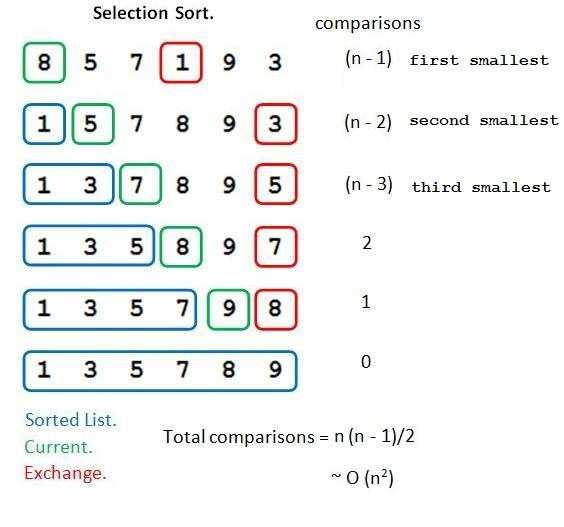（圖片來源：http://cheetahonfire.blogspot.sg/2009/05/selection-sort-vs-insertion-sort.html

const selectionSort = (arr) => {
const length = arr.length;

// 有幾個元素，就要找幾輪的最小值
// 這邊的 i 代表 i 以前的元素都排序好了
for (let i = 0; i < length; i++) {

// 先預設第一個是最小的
let min = arr[i];
let minIndex = i;

// 從還沒排好的元素開始找最小值
for (let j = i; j < length; j++) {
if (arr[j] < min) {
min = arr[j];
minIndex = j;
}
}

// ES6 的用法，交換兩個數值
[arr[minIndex], arr[i]] = [arr[i], arr[minIndex]];
}
return arr;
}


# 泡沫排序法（Bubble Sort）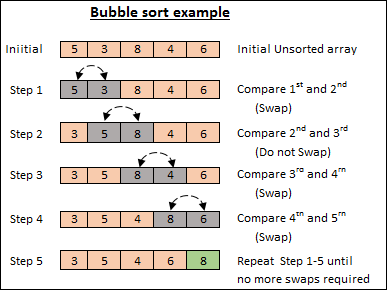（圖片來源：http://www.opentechguides.com/how-to/article/c/51/bubble-sort-c.html

const bubbleSort = (arr) => {
const n = arr.length;

// 一共要跑 n 輪
for (let i = 0; i < n; i++) {

// 從第一個元素開始，不斷跑到第 n - 1 - i 個
// 原本是 n - 1，會再加上 - i 是因為最後 i 個元素已經排好了
// 所以沒必要跟那些排好的元素比較
for (let j = 0; j < n - 1 - i; j++) {
if (arr[j] > arr[j + 1]) {
[arr[j], arr[j + 1]] = [arr[j + 1], arr[j]];
}
}
}

return arr;
}


function optimzedBubbleSort = (arr) => {
const  n = arr.length;
let swapped = true;

// 一共要跑 n 輪
for (let i = 0; i < n && swapped; i++) {

// 從第一個元素開始，不斷跑到第 n - 1 - i 個
// 原本是 n - 1，會再加上 - i 是因為最後 i 個元素已經排好了
// 所以沒必要跟那些排好的元素比較
swapped = false;
for (let j = 0; j < n - 1 - i; j++) {
if (arr[j] > arr[j + 1]) {
swapped = true;
[arr[j], arr[j + 1]] = [arr[j + 1], arr[j]];
}
}
}
return arr;
}


# 插入排序法（Insertion Sort）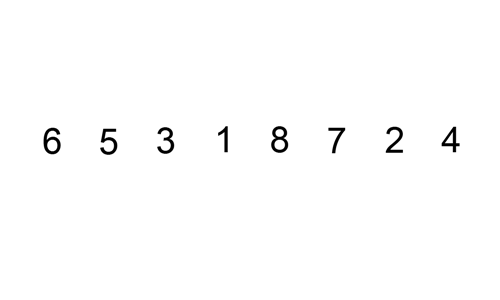（圖片來源：https://commons.wikimedia.org/wiki/File:Insertion-sort-example.gif

const insertionSort = (arr) => {
const n = arr.length;

// 假設第一個元素已經排好，所以從 1 開始跑
for (let i = 1; i < n; i++) {

// position 表示可以插入的位置
let position = i;

// 先把要插入的元素存起來
const value = arr[i];

// 開始往前找，只要符合這條件就代表這個位置是可以插入的
// 邊找的時候就可以邊把元素往後挪，騰出空間
while (i >= 0 && arr[position - 1] > value) {
[arr[position], arr[position - 1]] = [arr[position - 1], arr[position]];
position--;
}

// 找到適合的位置，放入元素
arr[position] = value;
}
return arr;
}


# 合併排序法（Merge Sort）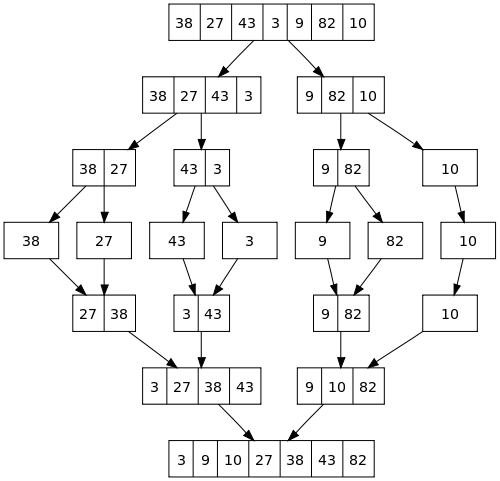（圖片來源：http://www.java2novice.com/java-sorting-algorithms/merge-sort/

const simpleMergeSort = (arr) => {

// 合併
const merge = (leftArray, rightArray) => {
let result = [];
let nowIndex = 0, left = 0, right = 0;
const leftLength = leftArray.length;
const rightLength = rightArray.length;

// 如果左右兩邊都沒抓完，就看誰比較小抓誰
while (left < leftLength && right < rightLength) {
if (leftArray[left] < rightArray[right]) {
result[nowIndex++] = leftArray[left++];
} else {
result[nowIndex++] = rightArray[right++];
}
}

// 跑到這裡代表左右兩邊其中一邊抓完了
// 如果是左邊沒抓完，全部抓下來
while (left < leftLength) {
result[nowIndex++] = leftArray[left++];
}

// 右邊沒抓完，全部抓下來
while (right < rightLength) {
result[nowIndex++] = rightArray[right++];
}

// 把合併好的陣列直接傳回去
return result;
}
const _mergeSort = (arr) => {
const length = arr.length;
if (length <= 1) return arr;

// 切兩半
const middle = Math.floor(length / 2);

// 排左邊
const leftArray = _mergeSort(arr.slice(0, middle));

// 排右邊
const rightArray = _mergeSort(arr.slice(middle, length));

// 合併後丟回去
return merge(leftArray, rightArray);
}
return _mergeSort(arr);
}


function mergeSort = (arr) => {
const merge = (array, start, middle, end) => {

// 宣告一個暫時的陣列來放合併後的結果
let temp = [];
let nowIndex = 0;
let left = start;
let right = middle + 1;

// 這邊都跟上面一樣
while (left <= middle && right <= end) {
if (array[left] < array[right]) {
temp[nowIndex++] = array[left++];
} else {
temp[nowIndex++] = array[right++];
}
}

while (left <= middle) {
temp[nowIndex++] = array[left++];
}

while (right <= end) {
temp[nowIndex++] = array[right++];
}

// 要把合併後的陣列放回去 array[start ~ end]
for (let i = start; i <= end; i++) {
array[i] = temp[i - start];
}
}

// 代表要從 start 排到 end
const _mergeSort = (array, start, end) => {
if (end <= start) return;
const middle = Math.floor((start + end) / 2);

// 對左右兩半排序
_mergeSort(array, start, middle);
_mergeSort(array, middle + 1, end);
merge(array, start, middle, end);
return array;
}
return _mergeSort(arr, 0, arr.length - 1);
}


# 快速排序法（Quick Sort）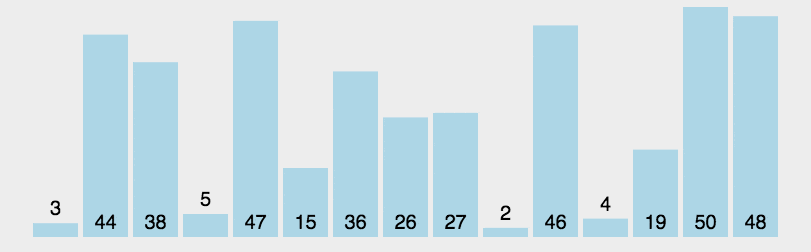（來源：https://github.com/hustcc/JS-Sorting-Algorithm/blob/master/6.quickSort.md

function quickSort = (arr) => {
const swap = (array, i , j) => {
[array[i], array[j]] = [array[j], array[i]];
}
const partition = (array, start, end) => {
let splitIndex = start + 1;
for (let i = start + 1; i <= end; i++) {
if (array[i] < array[start]) {
swap(array, i, splitIndex);
splitIndex++;
}
}

// 記得把 pivot 跟最後一個比它小的元素互換
swap(array, start, splitIndex - 1);
return splitIndex - 1;
}
const _quickSort = (array, start, end) => {
if (start >= end) return array;

// 在 partition 裡面調整數列，並且回傳 pivot 的 index
const middle = partition(array, start, end);
_quickSort(array, start, middle - 1);
_quickSort(array, middle + 1, end);
return array;
};
return _quickSort(arr, 0, arr.length - 1);
}


# 堆排序（Heap Sort）

Heap 是一種資料結構，並且有分兩種：max heap 跟 min heap，兩種的原理其實雷同，我們直接拿 max heap 來講。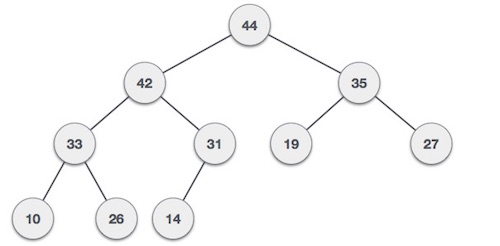（資料來源：https://www.tutorialspoint.com/data_structures_algorithms/heap_data_structure.htm

1. 父節點一定大於子節點
2. 整個樹的根節點一定是最大值（可以由 1 推出來）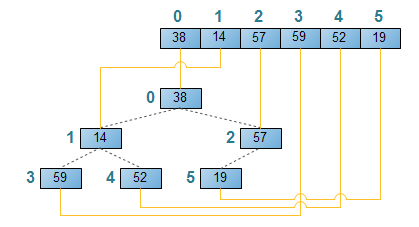（資料來源：http://notepad.yehyeh.net/Content/Algorithm/Sort/Heap/Heap.php

1. 先把讀進來的陣列建成 max heap（這時候 arr 一定是這陣列最大值）
2. 把 arr 跟最後一個節點互換（其實是最後一個還沒排序過的節點）
3. 調整成 max heap，回到步驟 2

heap sort 其實有點複雜，複雜到可以再獨立出來一篇了...

function heapSort = (arr) => {
function heapify(arr, length, node) {
const left = node * 2 + 1;
const right = node * 2 + 2;

// 先預設最大的節點是自己
let max = node;

if (left < length && arr[left] > arr[max]) {
max = left;
}

if (right < length && arr[right] > arr[max]) {
max = right;
}

// 如果左右兩邊有任何一個比 node 大的話
if (max !== node) {
// 就把兩個互換
[arr[node], arr[max]] = [arr[max], arr[node]];

// 接著繼續 heapfiy
heapify(arr, length, max);
}
}

// build max heap
const length = arr.length;
for (let i = Math.floor(length / 2) - 1; i>=0; i--) {
heapify(arr, length, i);
}

// 排序
for (let i = length - 1; i > 0; i--) {
[arr, arr[i]] = [arr[i], arr];
heapify(arr, i, 0);
}
return arr;
}


# 參考資料

@huli 野生工程師，相信分享與交流能讓世界變得更美好